Courses
Courses for Kids
Free study material
Offline Centres
More

# Tables and Shares Class 4 Notes CBSE Maths Chapter 11 (Free PDF Download)Last updated date: 29th Nov 2023
Total views: 163.5k
Views today: 4.63k

## Class 4 Chapter 11 Maths Revision Notes and Worksheets

Class 4 Maths syllabus is quite interesting in terms of introducing new concepts. You will use number table knowledge to understand the concepts in this chapter. Here, the questions with pictorial illustrations will be fascinating to solve and help to understand the new mathematical principles. Download and refer to the Tables and Shares revision notes developed by our subject experts from Vedantu to get a better understanding of this chapter.

These revision notes offer an easy explanation of tables, rows, columns, etc. Refer to these notes and learn how to solve the problems of multiplication and division using the knowledge of tables.

## Revision Notes Class 4 Mathematics Chapter 11 Tables and Shares

### Tables and Shares

• Tables refer to the multiplication of numbers.

• Shares refer to the division of two numbers.

### Multiplication

• Multiplication is linked to the grouping of objects.

• Example: Arrangement of children in the assembly hall, arrangement of books on the shelf, etc.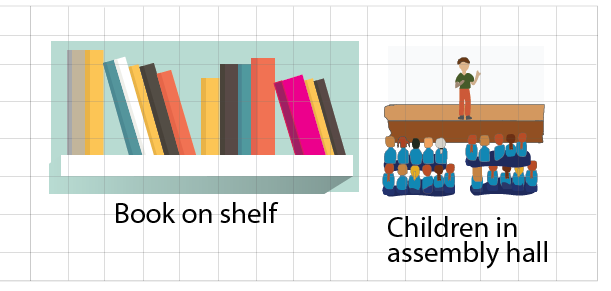Image:  Arrangement of children in the assembly hall, arrangement of books on the shelf

Solved Example 1: Can you arrange chairs in your classroom?

Ans: Yes, chairs in the classroom can be arranged.

### Arrangement Using Multiplication

• Multiplication can be used to arrange different items in rows.

• Arrange things in the form of rows and put an equal number of things in each row.

• So, by arranging an equal number of things in rows, we can find the total number of the objects

Solved Example 1: Help Sham in calculating the total number of apples in his bag.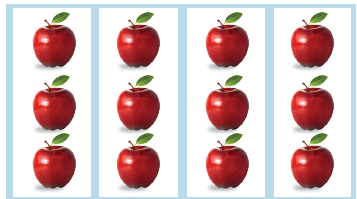Image: Apples in the bag

Ans: Sham has arranged apples in 3 rows. Here, Sham added 4 apples in each row.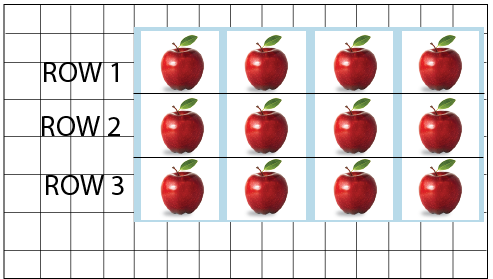Image: Sham has arranged apples in 3 rows

Step1: Create rows and add an equal number of apples in each row.

Step2: Write the number of rows = 3

Step3: Write the number of apples in each row = 4

Step4: Total number of apples = number of rows x number of apples in each row = 3 x 4 =12

So, Total number of apples = 3 x 4 =12

So, Sham has a total of 12 apples in his bag.

Solved Example 2: Riya has placed balls in her room in 3 rows, help Riya in finding the total balls with her?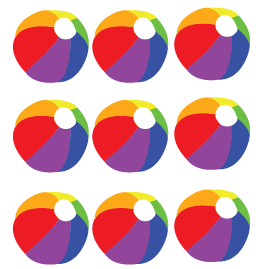Image: Riya placing balls in the room

Ans: Riya has arranged balls in 3 rows. Here, Riya added 3 balls in each row.

Step1: Write the number of rows = 3

Step2: Write the number of balls in each row = 3

Step3: Total number of balls = number of rows x number of balls in each row = 3 x 3 =9  So, Riya has a total of 9 balls in her room.

### Building Tables for Larger Numbers

• Tables for larger numbers are difficult to learn.

• A table of a larger number can be built by breaking a larger number into two smaller numbers.

• Note: The sum of two smaller numbers should be equal to the larger number.

• For example, let us create a table of 6. We can write 6 as (1+5), (2+4), and  (3+3). So, we can create a table of 6 by adding tables of (1 and 5), (2 and 4), or (3 and 3).

Solved Example 1: Let us create a table of 8 is created by using tables of 3 and 5.

Ans: Here, when 3 is added to 5 gives us 8, so we can use tables of 3 and 5 to get a table of 8.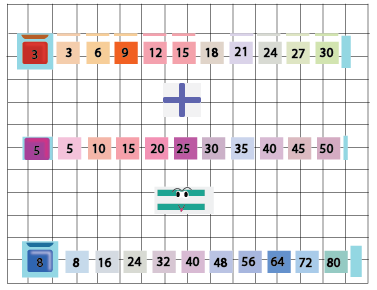Image: Table of 8 using tables 3 and 5

• Step1: Write tables of 3 and 5 in your notebooks.

• Step2: Start adding tables 3 and 5.

• Step3: After adding tables 3 and 5, the resultant table will be 8.

• Like, 3+5=8, 6+10=16, 9+15=24…………30+50=80.

• Similarly, we can create tables of various other numbers without memorizing them.

Solved Example 2: Can we create a Table of 8  by using tables of 2 and 6?

Ans: When 2 is added to 6 it gives us 8. So, yes we can create a table of 8 by adding tables of 2 and 6.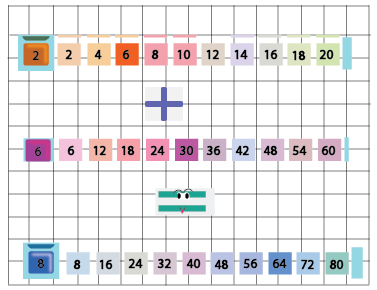Image: Table of 8 using tables 2 and 6

### Division

• The divide is to distribute something into equal parts.

• The division is linked to the fair sharing of things.

• Example: There are 15 chocolates and 3 friends want to share them. So, 15 is divided by 3 gives 5. So, each friend will get 5 chocolates.

Solved Example  1: Annie wants to distribute 12 candies equally among 3 children. How many candies will each child get?

Ans: Here, Annie wants to distribute 12 candies equally among 3 children. So, Annie will use the concept of division to find how many candies will each child get:

• Total number of candies Annie have= 12

• Total number of children = 3

• Candies are given to each child = Total number of candies÷Total number of children

• Candies are given to each child = 12 ÷ 3= 4

• So, each child will get 3 candies.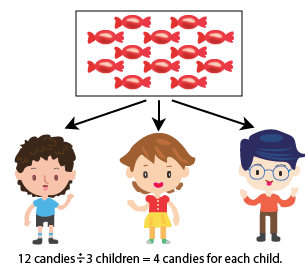Image: Annie distributing sweets among three children

Solved Example 2: Sam is participating in a 100-meter race. Sam jumps 5 steps at a time.Image: Sam participating in a race

Find (a) Count the jumps he needs to reach 90?

(b) How many jumps will he have taken if he is at 45?

(c) Sam reaches _____ after taking 7 jumps?

Ans: (a) Sam took 5 steps to complete 1 jump.

• Jumps taken by Sam to cover 5 steps = 1

• Jumps taken by Sam to cover 90 steps =90  ÷ 5 =18

• So, Sam has taken 18 jumps to reach 90.

(b) Sam took 5 steps to complete 1 jump.

• Jumps taken by Sam to cover 5 steps = 1

• Jumps taken by Sam to cover 45 steps = 45 ÷5 =9

• So, Sam has taken 9 jumps, if he is at 45.

(c) Sam took 5 steps to complete 1 jump.

• Steps were taken by Sam to cover 1 jump = 5

• Steps were taken by Sam to cover 7 jumps = 5 x 7 = 35

• So, Sam reaches 35 after taking 7 jumps.

### Division with Repeated Subtraction

• Subtracting a small number from a large number until the result is zero.

Solved Example 1: Jimmy has a total of 16 pineapples with her. She is packing 4 pineapples in each box. How many boxes does she need?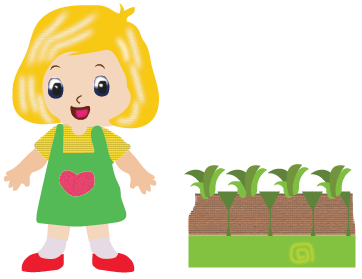Image: Jimmy packing pineapples

Ans: Total number of pineapples = 16

Number of pineapples in each box = 4

Hence, number of pineapples left after putting in 1st box = 16 - 4 = 12

number of pineapples left after putting in 2nd box = 12 - 4 = 8

number of pineapples left after putting in 3rd box = 8 - 4 = 4

number of pineapples left after putting in 4th box = 4 - 4 = 0

Thus, Jimmy needed 4 boxes to put 16 pineapples.

2: One carton can hold 100 soap bars. Reena wants to pack 300 soap bars. How many cartons does she need for packaging all of them?

Ans: Total number of soaps = 300

Number of soaps in each carton = 100

Hence, number of soaps left after putting in 1st carton = 300 - 100 = 200

number of soaps left after putting in 2nd carton = 200 - 100 = 100

number of soaps left after putting in 3rd carton = 100 - 100 = 0

Thus, Reena needed 3 cartons to put 300 soaps.

### Practice Problem

1. There are 90 mangoes. Distribute them equally in 10 baskets. How many mangoes will each basket contain?

Ans 1. Total number of mangoes = 90

Total number of baskets = 10

Mangoes in each basket = 90 ፥ 10 =9

So, there are 9 mangoes in each basket.

2. Jimmy packed 7 toys in each row in a wooden box that had 10 rows. Find the total number of toys Jimmy has?

Ans 2. Total number of rows = 10

Total number of toys in each row= 7

Total number of toys = 10 x 7 =70

So, Jimmy has 70 toys in the wooden box.

3. One carton can hold 200 soap bars. Meena wants to pack 1000 soap bars. How many cartons does she need for packaging all of them?

Ans 3: Total number of soaps = 1000

Number of soaps in each carton = 200

Hence, number of soaps left after putting in 1st carton = 1000 - 200 = 800

number of soaps left after putting in 2nd carton = 800 - 200 = 600

number of soaps left after putting in 3rd carton = 600 - 200 = 400

number of soaps left after putting in 4th carton = 400 - 200 = 200

number of soaps left after putting in 5th carton = 200 - 200 = 0

Thus, Meena needed 5 cartons to put 1000 soaps.

## Importance of CBSE Class 4 Maths Chapter 11 Tables and Shares

• This chapter teaches students how to use tables to solve problems. The sums given in this chapter emphasize using tables.

• Students will also learn to extract information from the problems and find the Tables and Shares Class 4 Solutions.

• They will also use the method of repetitive addition and subtraction and relate it to the tables they have studied in the previous classes.

• This chapter will also teach students how to read and understand arithmetic problems and solve them. They will learn to recognize the question patterns formulated by NCERT.

• Further progressing in this chapter, students will also learn to solve divisions and multiplications using tables of simple numbers. Hence, they will be able to develop concepts related to Tables and Shares accordingly.

## Tables and Shares Preparation Made Easier with Revision Notes and Worksheets

Follow how the experts have used the knowledge of tables to solve the sums given in this chapter. You can easily use the Maths 11 to 20 tables for solving the sums given in the exercises and worksheets. Develop excellent problem-solving skills by learning how to multiply and divide effectively with the methods given by the experts.

## Benefits of Using Vedantu for Class 4 Maths Chapter 11 Tables and Shares

Exploring Class 4 Maths Chapter 11 'Tables and Shares' with Vedantu offers young learners an interactive and comprehensive learning experience. With simplified concepts and engaging exercises, Vedantu's resources empower students to grasp multiplication, division, and the essence of shares effectively. These materials enhance mathematical proficiency, fostering a love for numbers in a fun and accessible manner. Here are a few Benefits of using Vedantu for Class 4 Maths Chapter 11 Tables and Shares:

• Vedantu's experts simplify this chapter's concepts for easy understanding.

• Connect your past learnings on addition, subtraction, multiplication, division, and number tables.

• The Tables and Shares PDF aids in quick chapter revision before exams.

• The concise presentation of sections minimizes time and effort during revisions.

• Practice with Class 4 Worksheets, comparing your answers with Vedantu's solutions for effective learning.

• Master the approach to solving multiplication problems, developing your unique problem-solving skills.

• Boost your exam scores with Vedantu's guidance in making math simple and enjoyable.

## Conclusion

For an enhanced comprehension of this subject, NCERT - Class 4 Maths Chapter 11 Tables and Shares thoughtfully prepared by experienced educators at Vedantu is your invaluable companion. These notes break down the complexities of Tables and Shares into easily digestible sections, helping you grasp new concepts master formulas, and navigate through questions effortlessly quickly in the last minute as well. By immersing yourself in these notes, you not only prepare for your studies more efficiently but also develop a profound understanding of the subject matter.

## FAQs on Tables and Shares Class 4 Notes CBSE Maths Chapter 11 (Free PDF Download)

1. Why are tables and shares important in Class 4 Maths Chapter 11?

Tables and shares teach fundamental concepts of multiplication and division, crucial for building mathematical skills.

2. How can I understand and memorize multiplication tables effectively Class 4 chapter 11?

Practice regularly, use visual aids, and engage in interactive learning methods to make memorization enjoyable and lasting.

3. What practical applications do tables and shares have in everyday life in class 4 chapter 11?

Understanding tables helps with calculations in daily tasks, and shares introduce the concept of fair distribution in real-life situations.

4. Are there any fun activities or games to reinforce the concepts of Tables and Shares chapter 11 class 4?

Yes, explore math games, group activities, and real-world scenarios to make learning tables and shares enjoyable and engaging.

5. How can parents support their child's learning of tables and shares at home in class 4 from chapter 11?

Encourage regular practice, provide real-life examples, and celebrate small achievements to foster a positive attitude towards math learning.